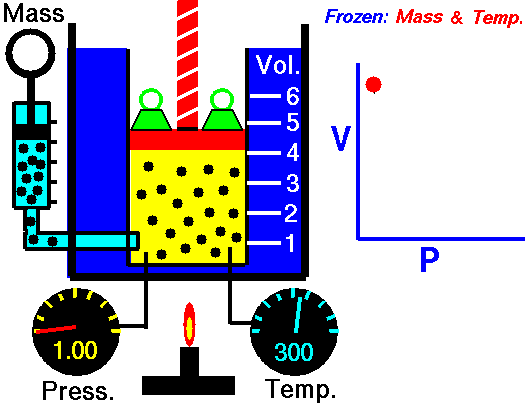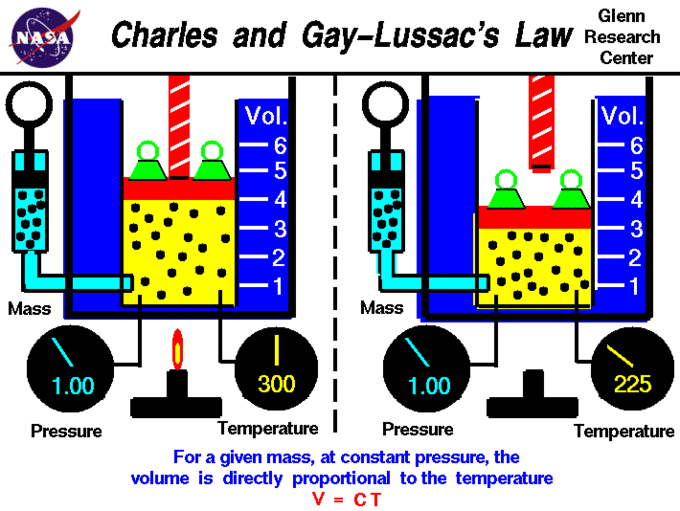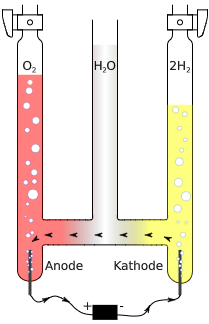## Boyle’s Law: Volume and Pressure

Boyle’s Law describes the inverse relationship between the pressure and volume of a fixed amount of gas at a constant temperature.

### Learning Objectives

Apply Boyle’s Law using mathematical calculations.

### Key Takeaways

#### Key Points

• According to Boyle’s Law, an inverse relationship exists between pressure and volume.
• Boyle’s Law holds true only if the number of molecules (n) and the temperature (T) are both constant.
• Boyle’s Law is used to predict the result of introducing a change in volume and pressure only, and only to the initial state of a fixed quantity of gas.
• The relationship for Boyle’s Law can be expressed as follows: P1V1 = P2V2, where P1 and V1 are the initial pressure and volume values, and P2 and V2 are the values of the pressure and volume of the gas after change.

#### Key Terms

• isotherm: in thermodynamics, a curve on a p-V diagram for an isothermal process
• Boyle’s law: the absolute pressure and volume of a given mass of confined gas are inversely proportional, while the temperature remains unchanged within a closed system
• ideal gas: a theoretical gas composed of a set of randomly-moving, non-interacting point particles

### Boyle’s Law

Boyle’s Law (sometimes referred to as the Boyle-Mariotte Law) states that the absolute pressure and volume of a given mass of confined gas are inversely proportional, provided the temperature remains unchanged within a closed system. This can be stated mathematically as follows:

$P_1V_1=P_2V_2$

### History and Derivation of Boyle’s Law

The law was named after chemist and physicist Robert Boyle, who published the original law in 1662. Boyle showed that the volume of air trapped by a liquid in the closed short limb of a J-shaped tube decreased in exact proportion to the pressure produced by the liquid in the long part of the tube.Boyle’s Law: An animation of Boyle’s Law, showing the relationship between volume and pressure when mass and temperature are held constant.

The trapped air acted much like a spring, exerting a force opposing its compression. Boyle called this effect “the spring of the air” and published his results in a pamphlet with that title. The difference between the heights of the two mercury columns gives the pressure (76 cm = 1 atm), and the volume of the air is calculated from the length of the air column and the tubing diameter.

The law itself can be stated as follows: for a fixed amount of an ideal gas kept at a fixed temperature, P (pressure) and V (volume) are inversely proportional—that is, when one doubles, the other is reduced by half.

Remember that these relations hold true only if the number of molecules (n) and the temperature (T) are both constant.

Interactive: The Volume-Pressure Relationship: Gases can be compressed into smaller volumes. How does compressing a gas affect its pressure? Run the model, then change the volume of the containers and observe the change in pressure. The moving wall converts the effect of molecular collisions into pressure and acts as a pressure gauge. What happens to the pressure when the volume changes?

### Example

In an industrial process, a gas confined to a volume of 1 L at a pressure of 20 atm is allowed to flow into a 12-L container by opening the valve that connects the two containers. What is the final pressure of the gas?

Set up the problem by setting up the known and unknown variables. In this case, the initial pressure is 20 atm (P1), the initial volume is 1 L (V1), and the new volume is 1L + 12 L = 13 L (V2), since the two containers are connected. The new pressure (P2) remains unknown.

P1V1 = P2V2

(20 atm)(1 L) = (P2)(13 L)

20 atom = (13) P2

P2 = 1.54 atm

The final pressure of the gas is 1.54 atm.

Boyle: An introduction to the relationship between pressure and volume, and an explanation of how to solve gas problems with Boyle’s Law.

## Charles’ and Gay-Lussac’s Law: Temperature and Volume

Charles’ and Gay-Lussac’s Law states that at constant pressure, temperature and volume are directly proportional.

### Learning Objectives

State Charles’ Law and its underlying assumptions

### Key Takeaways

#### Key Points

• The lower the pressure of a gas, the greater its volume (Boyle’s Law); at low pressures, $\frac{V}{273}$ will have a larger value.
• Charles’ and Gay-Lussac’s Law can be expressed algebraically as $\frac{\Delta V}{\Delta T} = \text{constant}$ or $\frac{V_1}{T_1} = \frac{V_2}{T_2}$.

#### Key Terms

• Charles’ law: at constant pressure, the volume of a given mass of an ideal gas increases or decreases by the same factor as its temperature on the absolute temperature scale (i.e. gas expands as temperature increases)
• absolute zero: the theoretical lowest possible temperature; by international agreement, absolute zero is defined as 0 K on the Kelvin scale and as −273.15° on the Celsius scale

### Charles’ and Guy-Lussac’s Law

Charles’ Law describes the relationship between the volume and temperature of a gas. The law was ﬁrst published by Joseph Louis Gay-Lussac in 1802, but he referenced unpublished work by Jacques Charles from around 1787. This law states that at constant pressure, the volume of a given mass of an ideal gas increases or decreases by the same factor as its temperature (in Kelvin); in other words, temperature and volume are directly proportional. Stated mathematically, this relationship is:

$\frac {V_1}{T_1}=\frac{V_2}{T_2}$

Interactive: The Temperature-Volume Relationship: This model contains gas molecules on the left side and a barrier that moves when the volume of gas expands or contracts, keeping the pressure constant. Run the model and change the temperature. Why does the barrier move when the temperature changes? (Note: Although the atoms in this model are in a flat plane, volume is calculated using 0.1 nm as the depth of the container.)

### Example

• A car tire filled with air has a volume of 100 L at 10°C. What will the expanded volume of the tire be after driving the car has raised the temperature of the tire to 40°C?
• $\frac {V_1}{T_1}=\frac{V_2}{T_2}$
• $\frac {\text{100 L}}{\text{283 K}}=\frac{V_2}{\text{313 K}}$
• $V_2=\text{110 L}$

### V vs. T Plot and Charles’ Law

A visual expression of Charles’ and Gay-Lussac’s Law is shown in a graph of the volume of one mole of an ideal gas as a function of its temperature at various constant pressures. The plots show that the ratio $\frac{V}{T}$ (and thus $\frac{\Delta V}{\Delta T}$) is a constant at any given pressure. Therefore, the law can be expressed algebraically as $\frac{\Delta V}{\Delta T} = \text{constant}$ or $\frac{V_1}{T_1} = \frac{V_2}{T_2}$.Charles’ and Gay–Lussac’s Law: A visual expression of the law of Charles and Gay-Lussac; specifically, a chart of the volume of one mole of an ideal gas as a function of its temperature at various constant pressures.

### Extrapolation to Zero Volume

If a gas contracts by 1/273 of its volume for each degree of cooling, it should contract to zero volume at a temperature of –273°C; this is the lowest possible temperature in the universe, known as absolute zero. This extrapolation of Charles’ Law was the first evidence of the significance of this temperature.

### Why Do the Plots for Different Pressures Have Different Slopes?

The lower a gas’ pressure, the greater its volume (Boyle’s Law), so at low pressures, the fraction $\frac{V}{273}$ will have a larger value; therefore, the gas must “contract faster” to reach zero volume when its starting volume is larger.

Charles: Discusses the relationship between volume and temperature of a gas, and explains how to solve problems using Charles’ Law.

## Avogadro’s Law: Volume and Amount

Avogadro’s Law states that at the same temperature and pressure, equal volumes of different gases contain an equal number of particles.

### Learning Objectives

State Avogadro’s Law and its underlying assumptions

### Key Takeaways

#### Key Points

• The number of molecules or atoms in a specific volume of ideal gas is independent of size or the gas’ molar mass.
• Avogadro’s Law is stated mathematically as follows: $\frac{V}{n} = k$, where V is the volume of the gas, n is the number of moles of the gas, and k is a proportionality constant.
• Volume ratios must be related to the relative numbers of molecules that react; this relationship was crucial in establishing the formulas of simple molecules at a time when the distinction between atoms and molecules was not clearly understood.

#### Key Terms

• Avogadro’s Law: under the same temperature and pressure conditions, equal volumes of all gases contain the same number of particles; also referred to as Avogadro’s hypothesis or Avogadro’s principle

Avogadro’s Law (sometimes referred to as Avogadro’s hypothesis or Avogadro’s principle) is a gas law; it states that under the same pressure and temperature conditions, equal volumes of all gases contain the same number of molecules. The law is named after Amedeo Avogadro who, in 1811, hypothesized that two given samples of an ideal gas—of the same volume and at the same temperature and pressure—contain the same number of molecules; thus, the number of molecules or atoms in a specific volume of ideal gas is independent of their size or the molar mass of the gas. For example, 1.00 L of N2 gas and 1.00 L of Cl2 gas contain the same number of molecules at Standard Temperature and Pressure (STP).

Avogadro’s Law is stated mathematically as:

$\frac{V}{n} = k$

V is the volume of the gas, n is the number of moles of the gas, and k is a proportionality constant.

As an example, equal volumes of molecular hydrogen and nitrogen contain the same number of molecules and observe ideal gas behavior when they are at the same temperature and pressure. In practice, real gases show small deviations from the ideal behavior and do not adhere to the law perfectly; the law is still a useful approximation for scientists, however.

Interactive: The Number-Volume Relationship: The model contains gas molecules under constant pressure. The barrier moves when the volume of gas expands or contracts. Run the model and select different numbers of molecules from the drop-down menu. What is the relationship between the number of molecules and the volume of a gas? (Note: Although the atoms in this model are in a flat plane, volume is calculated using 0.1 nm as the depth of the container.)

Early chemists calculated the molecular weight of oxygen using the incorrect formula HO for water. This lead to the molecular weight of oxygen being miscalculated as 8, rather than 16. However, when chemists found that an assumed reaction of H + Cl $\rightarrow$ HCl yielded twice the volume of HCl, they realized hydrogen and chlorine were diatomic molecules. The chemists revised their reaction equation to be H2 + Cl2 $\rightarrow$ 2HCl.
When chemists revisited their water experiment and their hypothesis that $HO \rightarrow H + O$, they discovered that the volume of hydrogen gas consumed was twice that of oxygen. By Avogadro’s Law, this meant that hydrogen and oxygen were combining in a 2:1 ratio. This discovery led to the correct molecular formula for water (H2O) and the correct reaction $2H_2O \rightarrow 2H_2 + O_2$.